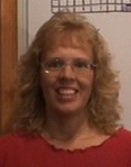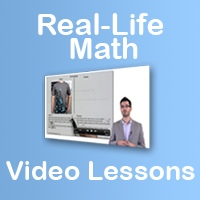# Maria's Math News, Vol. 53, May 2011

I love teaching, and I love math. This newsletter is my way of reaching out and helping you to teach it, too.1. Math Mammoth news & sale!
2. How to help a student with a fraction word problem
3. Decimals videos
4. Math vocabulary resource
5. Two problems about fractional parts
6. Tidbits

## 1. Math Mammoth news & sale!

• I will run a 20% off end-of-school year sale in the latter part of May, which will include all downloadable products & CDs, not printed books. STAY TUNED! I will send a separate email later.

• Suricate Publishing is the name of the company in South Africa that will publish a South African version of my books. This process has been slower than expected, but the plan is to go to print this week with first grade books... If you'd like to take a peek, see the website Suricate Publishing.

## 2. How to help a student with a fraction word problem

Today, my daughter had to tackle this word problem in her 5th grade Math Mammoth (lesson Multiply Mixed Numbers):The square on the right measures 10 in. × 10 in. and the rectangle inside it measures 6 7/8 in. × 3 1/2 in. How many square inches is the colored area?
This requires the student to
• multiply mixed numbers,
• subtract mixed numbers,
• understand how to find the "colored" area by subtraction of areas.
So it is a multi-step word problem.

She didn't understand it, she said. My first "help" was this: "Let's say we change those fractions to whole numbers 6 and 3. Can you mark those in the image? Would you be able to solve the problem now?"

The strategy I used is:
If you can't solve the problem at hand, change it and make it easier. Then try to solve the easier problem.Make Math Fun and Engaging Today! MakeMathMore.com's Real-Life Math Series offers: Activities that show students where math is in THEIR lives Lesson plans, student worksheets, extensions, and instructional videos New Lesson added monthly and MORE!

## 3. Decimals videos

Here are some of my recent additions to Math Mammoth Youtube channel. - videos about decimal arithmetic.

Instead of clicking the individual links to see the videos on YouTube, you can also watch them all on my blog.

I explain the main principle in adding or subtracting decimals: we can add or subtract "as if" there was no decimal point IF the decimals have the same kind of parts--either tenths, hundredths, or thousandths. Many students have a misconception of thinking of the "part" after the decimal point as "plain numbers." Such students will calculate 0.7 + 0.05 = 0.12, which is wrong, and I explain why in the video.

Multiply decimals by whole numbers
I explain how to multiply decimals by whole numbers: think of your decimal as so many "tenths", "hundredths", or "thousandths", and simply multiply as if there was no decimal point. Compare to multiplying so many "apples". For example, 5 x 0.06 is five copies of six "hundredths". Multiply 5 x 6 = 30. The answer has to be 30 hundredths (hundredths corresponding to apples), or 0.30, which simplifies to 0.3.
Multiply decimals by whole numbers video

Divide decimals using mental math
I explain two basic situations where you can use mental math to divide decimals: 1) Think of "stuff" (which is tenths, hundredths, or thousandths) shared evenly between so many people; OR 2) Think how many times the divisor fits into the dividend.
Divide decimals using mental math - video

Long division with decimals
When the dividend is a decimal, and the divisor is a whole number, long division is easy: just divide as if there was no decimal point, and then put a decimal point in the answer in the same place as it is in the dividend. I also show an example where we add decimal zeros to the dividend, in order to get an even division. Lastly I show how the fraction 3/7 is converted into a decimal using long division.
Long division with decimals - video

## 4. Math vocabulary resource

Would you like to help children with their math vocabulary? SpellingCity has built a resource to address this: Math vocabulary spelling lists.

If you don't know SpellingCity, no matter what kind of spelling list you use, you can always practice the words in many ways: either just simple practice AND with several different games: MatchIt Sentences, Which Word (find which word correctly completes the sentence), sentence unscramble, hang mouse, word search, word unscramble, etc. Can't even list them all.

## 5. Two problems about fractional parts

Here are two word problems on fractions which someone couldn't solve.
There were 3/5 as many adults as children on a bus. At the next bus stop, 6 adults and 6 children boarded the bus. As a result, there were 2/3 as many adults as children on the bus. How many people were on the bus at first?
A solution using bar diagrams (Singapore style):
```children |----|----|----|----|----|

```

Then we have:
```children |----|----|----|----|----| +6

```
Here look at the difference of children and adults. There are two "blocks" more children than adults. We know the number of adults is 2/3 of the number of children... therefore the DIFFERENCE of two blocks must be 1/3 of the children.

## 6. Tidbits

• A woman without hands - inspiring!
An inspiring slideshow about Jessica Cox - a woman born without hands. She even learned to fly!

These newsletters have different kinds of puzzles and good word problems, including challenging ones. I'm currently using them with both of my girls as a once-a-week math enrichment material, and I can highly recommend them!

• A new template for my blog
Click and see!

• Green tea word problem
I have solve many problems like this one on my blog, but it never hurts to solve some more. This can be solved with algebra, using a chart.

• Las tablas de multiplicar
I have ventured to make one video in Spanish... it is about how to effectively teach and drill multiplication tables.

• Backwards math
Denise shares one of her cutests and most adorable blogposts when her daughter did "backwards math!"

Feel free to forward this issue to a friend/colleague! Subscribe here.

Till next time,
Maria Miller